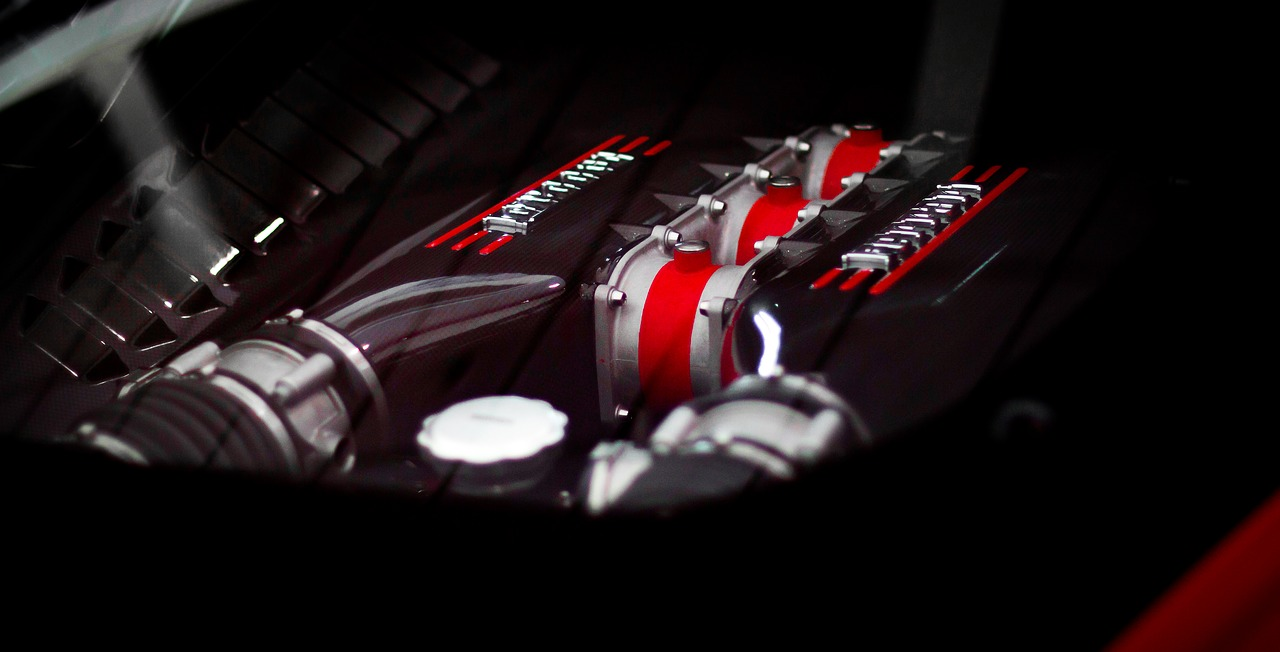Almost everybody has heard the term horsepower and most people generally understand what it means, but how is horsepower calculated exactly?

We dive into the details in this post and cover a bit of history at the same time.

When answering the question, how is horsepower calculated? We must first mention James Watt who invented the term. Watt is known for his work with steam engines and most noteworthy, lightbulbs.“The story goes that Watt was working with ponies lifting coal at a coal mine, and he wanted a way to talk about the power available from one of these animals. He found that, on average, a mine pony could do 22,000 foot-pounds of work in a minute. He then increased that number by 50 percent and pegged the measurement of horsepower at 33,000 foot-pounds of work in one minute. It is that arbitrary unit of measure that has made its way down through the centuries and now appears on your car, your lawn mower, your chain saw and even in some cases your vacuum cleaner.”

“What horsepower means is this: In Watt’s judgement, one horse can do 33,000 foot-pounds of work every minute. So, imagine a horse raising coal out of a coal mine as shown above. A horse exerting 1 horsepower can raise 330 pounds of coal 100 feet in a minute, or 33 pounds of coal 1,000 feet in one minute, or 1,000 pounds 33 feet in one minute. You can make up whatever combination of feet and pounds you like. As long as the product is 33,000 foot-pounds in one minute, you have a horsepower.”# Calculating And Converting Horsepower

It’s quite easy. Also, HP can be converted into other units of measurement. In the most basic sense, horsepower is a measurement for the rate at which work is done.

To calculate the horsepower of any machine you can use the equation:  Power = force× distance / time . You can also use a horsepower calculator.

One horsepower is roughly equivalent to 746 watts.

Consequently, one horsepower (over an hour) is equivalent to 2,545 BTU (British thermal units).

One BTU is equal to 1,055 joules, or 252 gram-calories or 0.252 food Calories.

# Dynamometers

A dynamometer (also called a dyno) is a tool for cars to test their horsepower. A dyno places a load on the engine and measures the amount of power that the engine can produce against the load. The numbers from a dynamometer reading will be measured in torque. The torque, aka the rotational equivalent of linear force (in pound-feet), needs to be converted to horsepower. To do the conversion, you simply multiply torque by rpm/5,252.